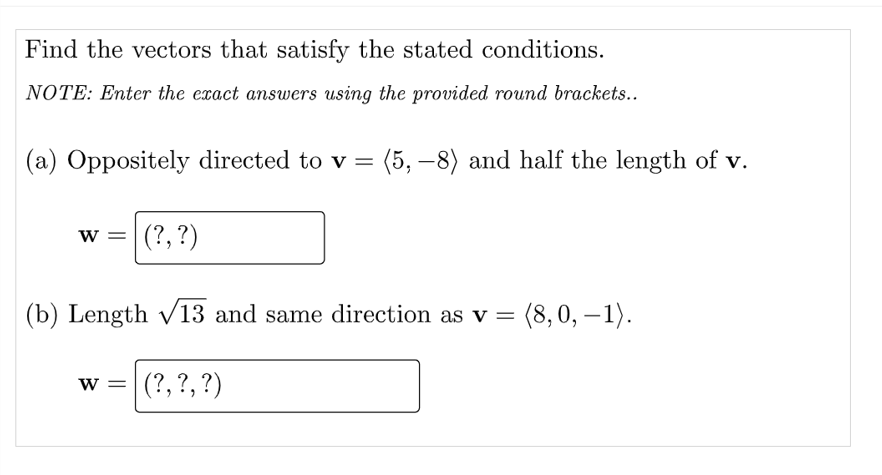# Question Find the vectors that satisfy the stated conditions. NOTE: Enter the ecact answers using the provided round brackets.. (a) Oppositely directed to v= : (5, -8) and half the length of v. w = |(?, ?) ) (b) Length V13 and same direction as v = (8,0, –1). = w=|(?, ?, ?) 7